# Linear Momentum, Angular Momentum and Kinetic Energy of Rigid Bodies (Part - 1) Civil Engineering (CE) Notes | EduRev

## Civil Engineering (CE) : Linear Momentum, Angular Momentum and Kinetic Energy of Rigid Bodies (Part - 1) Civil Engineering (CE) Notes | EduRev

The document Linear Momentum, Angular Momentum and Kinetic Energy of Rigid Bodies (Part - 1) Civil Engineering (CE) Notes | EduRev is a part of the Civil Engineering (CE) Course Introduction to Dynamics and Vibrations- Notes, Videos, MCQs.
All you need of Civil Engineering (CE) at this link: Civil Engineering (CE)

6.4 Linear momentum, angular momentum and kinetic energy of rigid bodies

In this section, we determine how to calculate the angular momentum and kinetic energy of a rigid body, and define two important quantities: (1) the center of mass of a rigid body (which you already know), and (2) the Inertia tensor (matrix) of a rigid body.

To keep things simple, we won’t consider a general rigid body right away. Instead, we will calculate the linear momentum, angular momentum, and kinetic energy of a system of N particles that are connected together by rigid, massless links.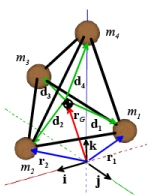Definitions of inertial properties: For this system, we will define

• The total mass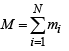• The position of the center of mass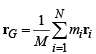• The position vector of each mass relative to the center of mass di = ri −rG
• The velocity of the center of mass VG = drG/dt
• The mass moment of inertia about the center of mass (a tensor, which can be expressed as a matrix if we choose a coordinate system and set di = dixi + diy j+ dizk )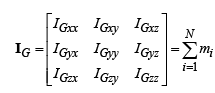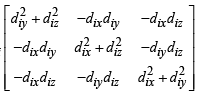The mass moment of inertia is sometimes also written in a more abstract but very compact way as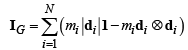Here, 1 is the identity tensor, and di ⊗di is a tensor with components dixdix , dixdiy , dixdiz , etc (the symbol ⊗ is called the ‘diadic product’ of two vectors).

Formulas for linear and angular momentum and kinetic energy: We will show that:

• The total linear momentum is p = MvG
• The total angular momentum (about the origin) is h =rG × MvG+ IGω
• The total kinetic energy is T = 1/2 mvG ·vG + 1/2 ω ·IGω

These are actually general results that hold for all rigid bodies, as long as we use a more general definition of M and IG

Simplified formulas for two dimensions: For planar problems, diz = 0 (since all the masses are in the plane), and ω = ωzk . In this case, we can use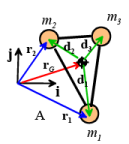• The total linear momentum is p = MvG
• The total angular momentum (about the origin) is h =rG × MvG+IGzzω z k
• The total kinetic energy is T = 1/2 MvG ·vG + 1/2 IGzzω2z

Here IGzz is just the bottom diagonal term of the full inertia matrix (i.e. just a single number)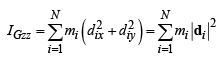Example 1: A simple 3D assembly of masses is shown in the figure.

(1) Find the mass moment of inertia.

By symmetry, the COM is at the origin. The inertia tensor is therefore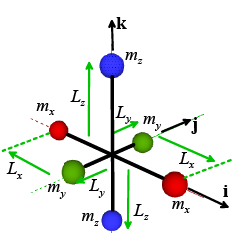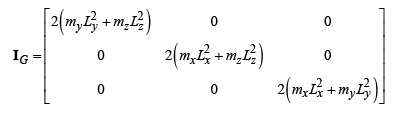(2) Assume that the COM is stationary (i.e. the assembly rotates about the origin). Find formulas for the angular momentum and kinetic energy of the system, in terms of the angular velocity components ωxyz

The formula gives the angular momentum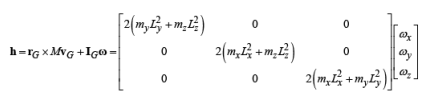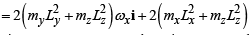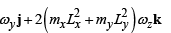Note that h is a vector. Importantly, h is not generally parallel to the angular velocity vector, as this example shows.

The kinetic energy is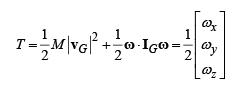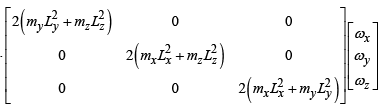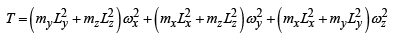These results help us understand what the formulas are predicting.  Note, for example, that:

• The mass moment of inertia always has the form mass*length2.   It has units of kg-m2
• The mass moment of inertia is a measure of how mass is distributed about the center of mass.  An object has a large inertia if the mass is far from the COM, and a small one if the mass is close to the COM.
• The matrix-vector products in the formulas for h and T are really just a way of calculating the velocity of each particle in the system in a quick way. For example, suppose we rotate our assembly of masses about the k axis with angular velocity ωz (see the animation).Let’s calculate the kinetic energy of the system, but without using the rigid body formulas.  The two blue masses are stationary, so they have no KE. The red and green mass are both moving in a circle about the origin. The circular motion formula says their speed is V = Rωz We can calculate the total kinetic energy using the usual formula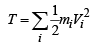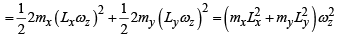This is the same result we calculated with the rigid body formula.

This explains why the formula for IGzz contains Lx and Ly - the IGzz component keeps track of how much energy or momentum is produced by a rotation about the z axis.   The energy and momentum depend on the distances of the masses from the z axis – which of course depends on Lx and Ly.

Finally, note that we can interpret the two terms in the formulas for momentum and KE as quantifying (separately) the effects of translation and rotation

h = rG × MvG + IGω

Angular momentum Translational + Rotational

Kinetic energy is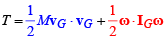Translational + Rotational

This helps explain why we can often idealize a system as a particle.  If the rotational term is negligible, the angular momentum and kinetic energy of a rigid body is just the same as that of a particle located at the COM.

Offer running on EduRev: Apply code STAYHOME200 to get INR 200 off on our premium plan EduRev Infinity!

## Introduction to Dynamics and Vibrations- Notes, Videos, MCQs

20 videos|53 docs

,

,

,

,

,

,

,

,

,

,

,

,

,

,

,

,

,

,

,

,

,

,

,

,

;